Miscellaneous

Practical Geometry
Serial order wise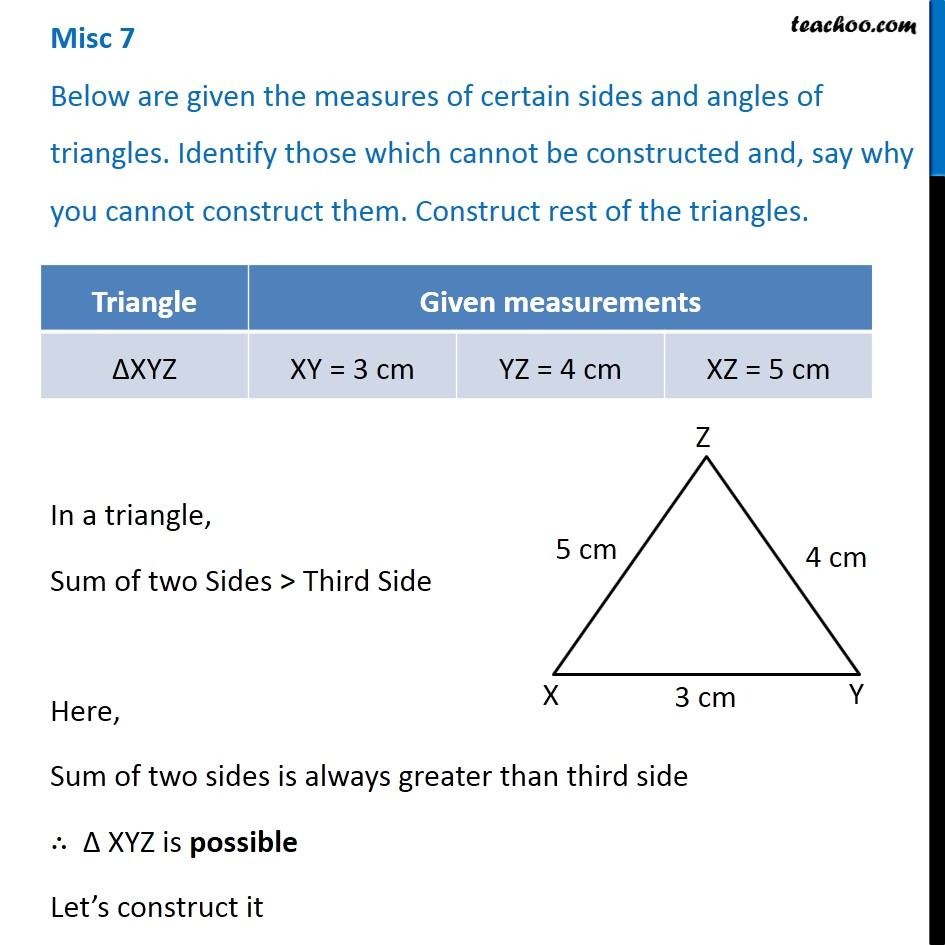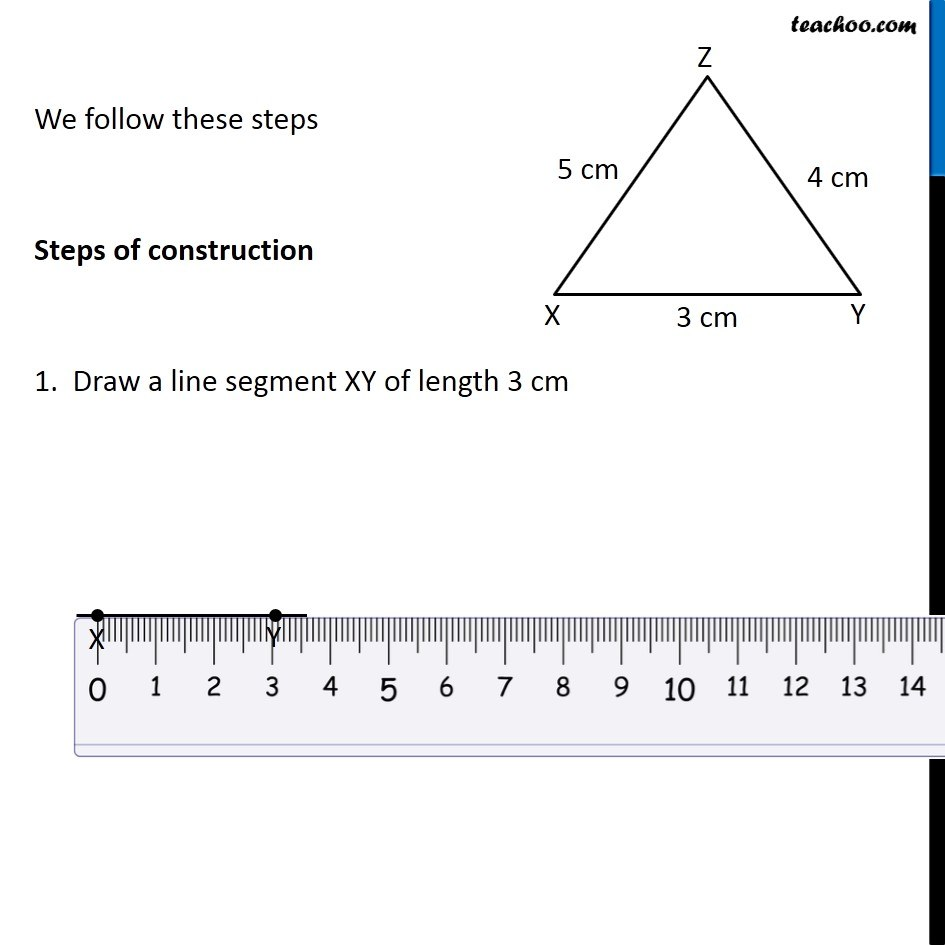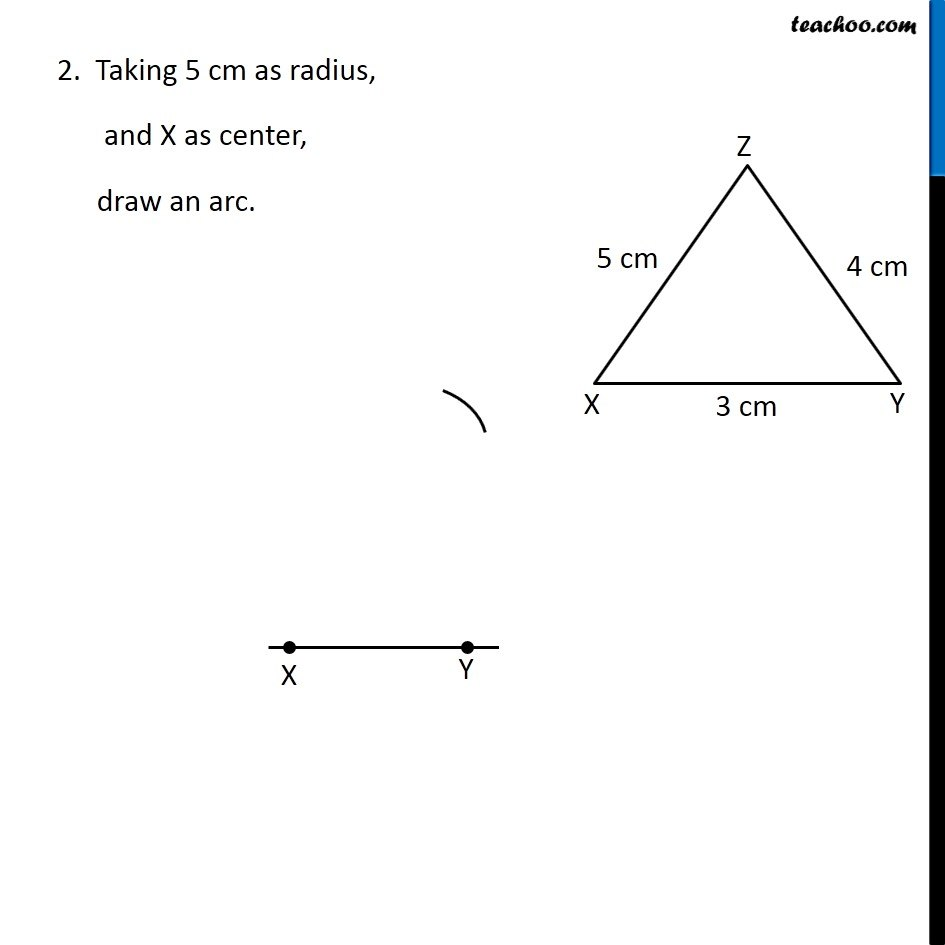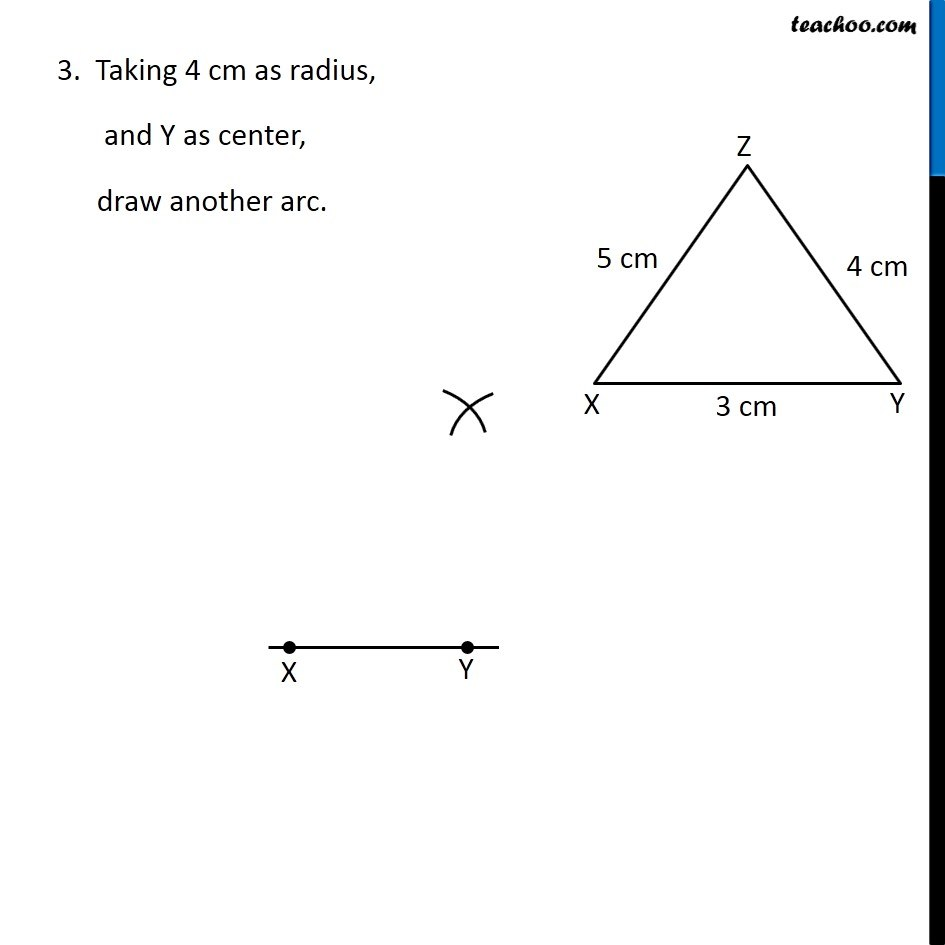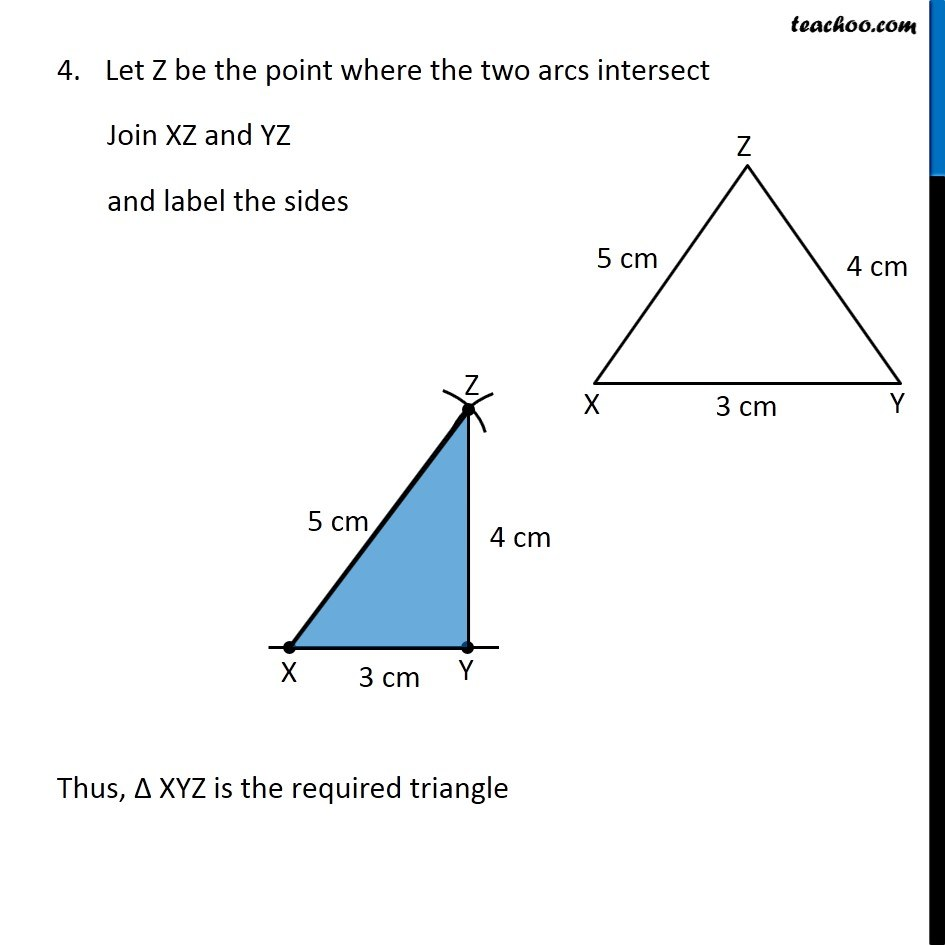Learn in your speed, with individual attention - Teachoo Maths 1-on-1 Class

### Transcript

Misc 7 Below are given the measures of certain sides and angles of triangles. Identify those which cannot be constructed and, say why you cannot construct them. Construct rest of the triangles. In a triangle, Sum of two Sides > Third Side Here, Sum of two sides is always greater than third side ∴ Δ XYZ is possible Let’s construct it We follow these steps Steps of construction 1. Draw a line segment XY of length 3 cm 2. Taking 5 cm as radius, and X as center, draw an arc. 3. Taking 4 cm as radius, and Y as center, draw another arc. Let Z be the point where the two arcs intersect Join XZ and YZ and label the sides Thus, Δ XYZ is the required triangle